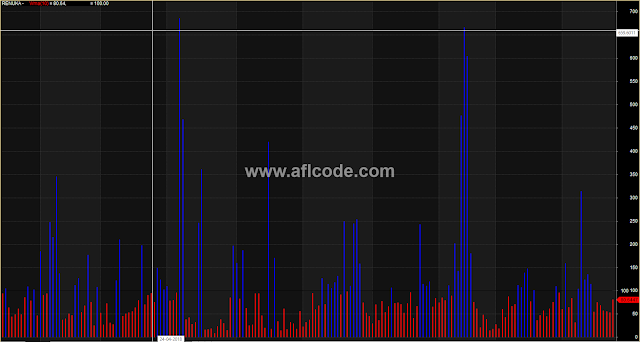### Volume Compared With Moving AverageVolume Compared With Moving Average

```//www.aflcode.com

pds = 10;
V1 = V/MA(V,10); V2 = V/MA(V,20);
V3 = V/MA(V,50);

barcolor = IIf(V<MA(V,pds),4,6);
/* Colourized price bars drawn here */
Plot(100*Volume/MA(V,pds),"    V/ma(10)",barcolor,2+4);

Plot(100,"    AVG(10)",1,1);

unsure = V1<1 AND V2<1 AND V3<1;
sure   = V1>1 AND V2>1 AND V3>1;

Filter = unsure;